• # 返回数据的极大似然估计，估计出参数约为30和2 参考文献 Statistics (scipy.stats) — SciPy v1.4.1 Reference Guide Scipy：高级科学计算 | SciPy Lecture Notes 中文版 极大似然估计MLE原理+python实现 ...
概念
现实中任何随机变量的概率分布函数都是未知的。
如果假定随机变量服从某种分布（如正态分布），可以通过统计手段来计算该分布的参数，这种方法称为参数估计。
极大似然估计（Maximum Likelihood Estimate, MLE）是一种参数估计的方法，利用已知样本结果，反推最大可能导致这样结果的参数值。安装
pip install scipy

代码
若随机变量X服从一个数学期望为$μ$、方差为$σ^2$的正态分布，记为$N(μ,σ^2)$
import numpy as np
from scipy.stats import norm
import matplotlib.pyplot as plt

μ = 30  # 数学期望
σ = 2  # 方差
x = μ + σ * np.random.randn(10000)  # 正态分布
plt.hist(x, bins=100)  # 直方图显示
plt.show()
print(norm.fit(x))  # 返回极大似然估计，估计出参数约为30和2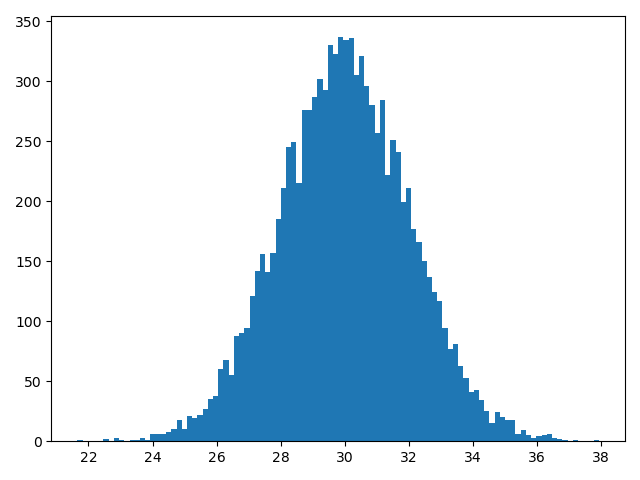参考文献

Statistics (scipy.stats) — SciPy v1.4.1 Reference Guide
Scipy：高级科学计算 | SciPy Lecture Notes 中文版
极大似然估计MLE原理+python实现
python简单实现最大似然估计&scipy库的使用
极大似然估计详解


展开全文机器学习
• ## 极大似然估计详解，写的太好了！

万次阅读 多人点赞 2018-08-18 15:42:08
极大似然估计  以前多次接触过极大似然估计，但一直都不太明白到底什么原理，最近在看贝叶斯分类，对极大似然估计有了新的认识，总结如下：   贝叶斯决策  首先来看贝叶斯分类，我们都知道经典的贝叶斯公式：...
极大似然估计

以前多次接触过极大似然估计，但一直都不太明白到底什么原理，最近在看贝叶斯分类，对极大似然估计有了新的认识，总结如下：

贝叶斯决策

首先来看贝叶斯分类，我们都知道经典的贝叶斯公式：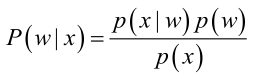其中：p(w)：为先验概率，表示每种类别分布的概率；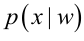：类条件概率，表示在某种类别前提下，某事发生的概率；而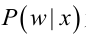为后验概率，表示某事发生了，并且它属于某一类别的概率，有了这个后验概率，我们就可以对样本进行分类。后验概率越大，说明某事物属于这个类别的可能性越大，我们越有理由把它归到这个类别下。

我们来看一个直观的例子：已知：在夏季，某公园男性穿凉鞋的概率为1/2，女性穿凉鞋的概率为2/3，并且该公园中男女比例通常为2:1，问题：若你在公园中随机遇到一个穿凉鞋的人，请问他的性别为男性或女性的概率分别为多少？

从问题看，就是上面讲的，某事发生了，它属于某一类别的概率是多少？即后验概率。

设：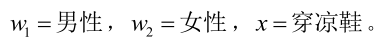由已知可得：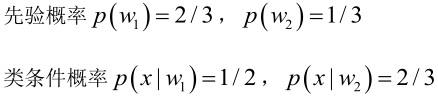男性和女性穿凉鞋相互独立，所以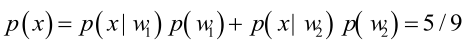（若只考虑分类问题，只需要比较后验概率的大小，的取值并不重要）。

由贝叶斯公式算出：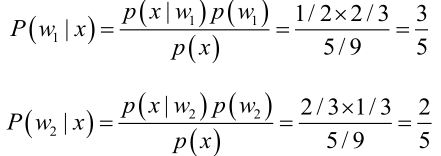问题引出

但是在实际问题中并不都是这样幸运的，我们能获得的数据可能只有有限数目的样本数据，而先验概率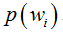和类条件概率(各类的总体分布)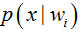都是未知的。根据仅有的样本数据进行分类时，一种可行的办法是我们需要先对先验概率和类条件概率进行估计，然后再套用贝叶斯分类器。

先验概率的估计较简单，1、每个样本所属的自然状态都是已知的（有监督学习）；2、依靠经验；3、用训练样本中各类出现的频率估计。

类条件概率的估计（非常难），原因包括：概率密度函数包含了一个随机变量的全部信息；样本数据可能不多；特征向量x的维度可能很大等等。总之要直接估计类条件概率的密度函数很难。解决的办法就是，把估计完全未知的概率密度转化为估计参数。这里就将概率密度估计问题转化为参数估计问题，极大似然估计就是一种参数估计方法。当然了，概率密度函数的选取很重要，模型正确，在样本区域无穷时，我们会得到较准确的估计值，如果模型都错了，那估计半天的参数，肯定也没啥意义了。

重要前提

上面说到，参数估计问题只是实际问题求解过程中的一种简化方法（由于直接估计类条件概率密度函数很困难）。所以能够使用极大似然估计方法的样本必须需要满足一些前提假设。

重要前提：训练样本的分布能代表样本的真实分布。每个样本集中的样本都是所谓独立同分布的随机变量 (iid条件)，且有充分的训练样本。

极大似然估计

极大似然估计的原理，用一张图片来说明，如下图所示：总结起来，最大似然估计的目的就是：利用已知的样本结果，反推最有可能（最大概率）导致这样结果的参数值。

原理：极大似然估计是建立在极大似然原理的基础上的一个统计方法，是概率论在统计学中的应用。极大似然估计提供了一种给定观察数据来评估模型参数的方法，即：“模型已定，参数未知”。通过若干次试验，观察其结果，利用试验结果得到某个参数值能够使样本出现的概率为最大，则称为极大似然估计。

由于样本集中的样本都是独立同分布，可以只考虑一类样本集D，来估计参数向量θ。记已知的样本集为：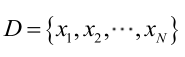似然函数（linkehood function）：联合概率密度函数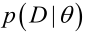称为相对于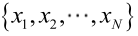的θ的似然函数。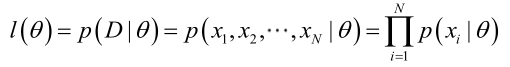如果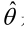是参数空间中能使似然函数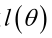最大的θ值，则应该是“最可能”的参数值，那么就是θ的极大似然估计量。它是样本集的函数，记作：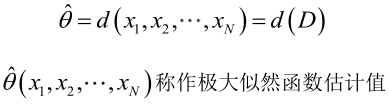求解极大似然函数

ML估计：求使得出现该组样本的概率最大的θ值。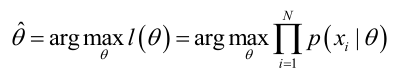实际中为了便于分析，定义了对数似然函数：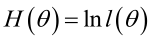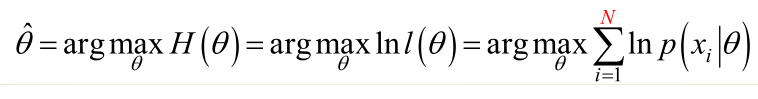1. 未知参数只有一个（θ为标量）

在似然函数满足连续、可微的正则条件下，极大似然估计量是下面微分方程的解：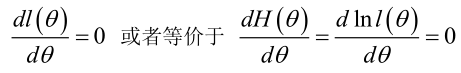2.未知参数有多个（θ为向量）

则θ可表示为具有S个分量的未知向量：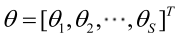记梯度算子：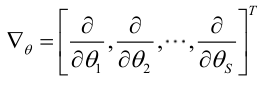若似然函数满足连续可导的条件，则最大似然估计量就是如下方程的解。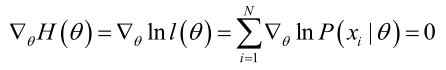方程的解只是一个估计值，只有在样本数趋于无限多的时候，它才会接近于真实值。

极大似然估计的例子

例1：设样本服从正态分布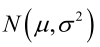，则似然函数为：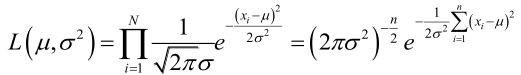它的对数：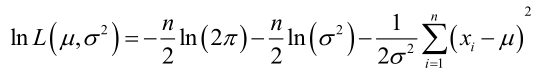求导，得方程组：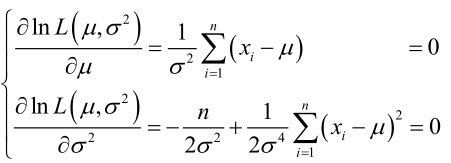联合解得：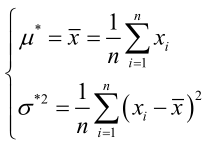似然方程有唯一解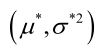：，而且它一定是最大值点，这是因为当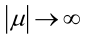或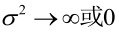时，非负函数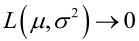。于是U和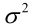的极大似然估计为。

例2：设样本服从均匀分布[a, b]。则X的概率密度函数：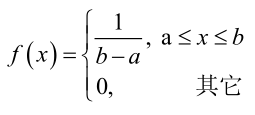对样本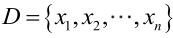：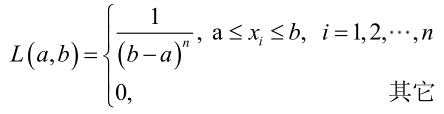很显然，L(a,b)作为a和b的二元函数是不连续的，这时不能用导数来求解。而必须从极大似然估计的定义出发，求L(a,b)的最大值，为使L(a,b)达到最大，b-a应该尽可能地小，但b又不能小于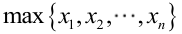，否则，L(a,b)=0。类似地a不能大过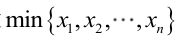，因此，a和b的极大似然估计：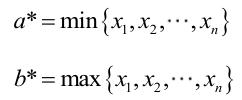总结

求最大似然估计量的一般步骤：

（1）写出似然函数；

（2）对似然函数取对数，并整理；

（3）求导数；

（4）解似然方程。

最大似然估计的特点：

1.比其他估计方法更加简单；

2.收敛性：无偏或者渐近无偏，当样本数目增加时，收敛性质会更好；

3.如果假设的类条件概率模型正确，则通常能获得较好的结果。但如果假设模型出现偏差，将导致非常差的估计结果。

正态分布ML估计的Matlab实例：点击打开链接

版权声明：本文为博主原创文章，未经博主允许不得转载。 https://blog.csdn.net/zengxiantao1994/article/details/72787849
展开全文线性回归

全栈工程师开发手册 （作者：栾鹏）

python数据挖掘系列教程

极大似然

极大似然（Maximum Likelihood）估计为用于已知模型的参数估计的统计学方法。

也就是求使得似然函数最大的代估参数的值。而似然函数就是如果参数已知则已出现样本出现的概率。

比如，我们想了解抛硬币是正面（head）的概率分布θ$\theta$$θ$；那么可以通过最大似然估计方法求得。

θ^=argmaxxl(θ)=argmaxxθ8(1−θ)2$\stackrel{^}{\theta }=argma{x}_{x}l\left(\theta \right)=argma{x}_{x}{\theta }^{8}\left(1-\theta {\right)}^{2}$\hat{θ} =argmax_x l(θ)=argmax_x θ^8 (1−θ)^2

其中，l(θ)$l\left(\theta \right)$$l(θ)$为观测变量序列的似然函数。

所以最大似然方法估计参数，就是先假设参数已知，然后用参数，求出样本出现的概率。如果是多个样本，就是多个样本的联合概率最大

求解使似然函数最大的代估参数，常规的做法就是对似然函数求导，求使导数为0的自变量的值，以及左右边界线的值。

例如

对l(θ)$l\left(\theta \right)$$l(θ)$求偏导

∂l(θ)∂θ=θ7(1−θ)(8−10θ)⇒θ^=0.8$\frac{\partial l\left(\theta \right)}{\partial \theta }={\theta }^{7}\left(1-\theta \right)\left(8-10\theta \right)⇒\stackrel{^}{\theta }=0.8$\frac{∂l(θ)}{∂θ} =θ^7 (1−θ)(8−10θ)⇒\hat{θ} =0.8

但是如果似然函数不是凹函数（concave），求解极大值困难。一般地，使用与之具有相同单调性的log-likelihood，如图所示也就是将似然函数求log。所谓的凹函数和凸函数，凹函数斜率逐渐减小，凸函数斜率逐渐增大。所以凹函数“容易”求解极大值（极值为0时），凸函数“容易”求解极小值（极值为0时）。

EM算法

EM算法（Expectation Maximization）是在含有隐变量（latent variable）的模型下计算最大似然的一种算法。所谓隐变量，是指我们没有办法观测到的变量。比如，有两枚硬币A、B，每一次随机取一枚进行抛掷，我们只能观测到硬币的正面与反面，而不能观测到每一次取的硬币是否为A；则称每一次的选择抛掷硬币为隐变量。

用Y表示观测数据，Z表示隐变量；Y和Z连在一起称为完全数据( complete-data )，观测数据Y又称为不完全数据(incomplete-data)。观测数据的似然函数：
P(Y|θ)=∑zP(Z|θ)P(Y|Z,θ)$P\left(Y|\theta \right)={\sum }_{z}P\left(Z|\theta \right)P\left(Y|Z,\theta \right)$P(Y|θ)=∑ _z P(Z|θ)P(Y|Z,θ)

求模型参数的极大似然估计：

θ^=argmaxθlogP(Y|θ)$\stackrel{^}{\theta }=argma{x}_{\theta }logP\left(Y|\theta \right)$\hat{θ}  =argmax_{θ} logP(Y|θ)

因为含有隐变量，此问题无法求解。因此，Dempster等人提出EM算法用于迭代求解近似解。

所以EM算法是一种特殊情况下的最大似然求解方法。

EM算法比较简单，分为两个步骤：

E步（E-step），以当前参数θ(i)${\theta }^{\left(i\right)}$$θ^{(i)}$ 计算Z$Z$$Z$的期望值。因为期望值中不再包含未知的隐含变量Z，所以是可以计算的。

Q(θ,θ(i))=EZ[logP(Y,X|θ)|Y,θ(i)]$Q\left(\theta ,{\theta }^{\left(i\right)}\right)={E}_{Z}\left[logP\left(Y,X|\theta \right)|Y,{\theta }^{\left(i\right)}\right]$Q(θ,θ^{(i)} )=E_Z [logP(Y,X|θ)|Y,θ^{(i)} ]

M步（M-step），求使Q(θ,θ(i))$Q\left(\theta ,{\theta }^{\left(i\right)}\right)$$Q(θ,θ^{(i)})$极大化的θ$\theta$$θ$，确定第i+1$i+1$$i+1$次迭代的参数的估计值θ(i+1)${\theta }^{\left(i+1\right)}$$θ^{(i+1)}$

θ(i+1)=argmaxθQ(θ,θ(i))${\theta }^{\left(i+1\right)}=argma{x}_{\theta }Q\left(\theta ,{\theta }^{\left(i\right)}\right)$θ^{(i+1)} =argmax_θ   Q(θ,θ^{(i)} )

如此迭代直至算法收敛。

案例

如图所示，有两枚硬币A、B，每一个实验随机取一枚抛掷10次，共5个实验，我们可以观测到每一次所取的硬币，估计参数A、B为正面的概率θ=(θA,θB)$\theta =\left({\theta }_{A},{\theta }_{B}\right)$$θ=(θ_A ,θ_B )$ ，根据极大似然估计求解。如果我们不能观测到每一次所取的硬币，只能用EM算法估计模型参数，算法流程如图所示：隐变量Z$Z$$Z$为每次实验中选择A或B的概率，并初始化A为正面的概率为0.6，B为正面的概率为0.5。实验进行了5次。每次都要进行一遍EM操作。每次都要计算隐含变量。取A的概率，和取B的概率。然后更新代估参数θA${\theta }_{A}$$θ_A$（A为正面的概率）和θB${\theta }_{B}$$θ_B$（B为正面的概率）的值。

在初始化θA=0.6${\theta }_{A}=0.6$$θ_A=0.6$和θB=0.5${\theta }_{B}=0.5$$θ_B=0.5$后第一次实验后计算隐含变量（选择A的概率）为

P(z1=A|y1,θ0)=z1为A的话，样本结果出现的概率z1为任何可取值的话，样本结果出现的概率=P(z1=A|y1,θ0)P(z1=A|y1,θ0)+P(z1=B|y1,θ0)=0.65∗0.450.65∗0.45+0.510=0.45$P\left({z}_{1}=A|{y}_{1},{\theta }^{0}\right)=\frac{{z}_{1}为A的话，样本结果出现的概率}{{z}_{1}为任何可取值的话，样本结果出现的概率}=\frac{P\left({z}_{1}=A|{y}_{1},{\theta }^{0}\right)}{P\left({z}_{1}=A|{y}_{1},{\theta }^{0}\right)+P\left({z}_{1}=B|{y}_{1},{\theta }^{0}\right)}=\frac{{0.6}^{5}\ast {0.4}^{5}}{{0.6}^{5}\ast {0.4}^{5}+{0.5}^{10}}=0.45$P(z_1 =A|y_1 ,θ^0)=\frac{z_1为A的话，样本结果出现的概率}{z_1为任何可取值的话，样本结果出现的概率}=\frac{P(z_1 =A|y_1 ,θ^0)}  { P(z_1 =A|y_1 ,θ^0)+P(z_1 =B|y_1 ,θ^0) }=\frac{0.6^5 ∗0.4^5}{0.6^5 ∗0.4^5 +0.5^{10}}  =0.45

按照上面的计算方法可依次求出其他隐含变量Z$Z$$Z$，然后计算极大化的θ(i)${\theta }^{\left(i\right)}$$θ^{(i)}$。经过10次迭代，最终收敛。

K均值聚类和EM算法

K均值聚类是无监督的聚类算法。
关于k均值聚类不了解的可以参考：https://blog.csdn.net/luanpeng825485697/article/details/78993977

k均值聚类的目的就是为了是下面的损失函数最小

J(c,u)=∑i=1m||xi−uci||$J\left(c,u\right)=\sum _{i=1}^{m}||{x}_{i}-{u}_{{c}_{i}}||$J(c,u)=\sum_{i=1}^m||x_i-u_{c_i}||

其中m为样本个数，xi${x}_{i}$$x_i$表示第i$i$$i$个样本，ci${c}_{i}$$c_i$表示第第i个样本所属的聚类，uci${u}_{{c}_{i}}$$u_{c_i}$表示第i个样本所属的聚类的质心。

假设当前J$J$$J$没有达到最小值，那么首先可以固定每个类的质心uj${u}_{j}$$u_j$，调整每个样例的所属的类别cj${c}_{j}$$c_j$来让J$J$$J$函数减少，同样，固定cj${c}_{j}$$c_j$，调整每个类的质心uj${u}_{j}$$u_j$也可以使J$J$$J$减小。这两个过程就是内循环中使J$J$$J$单调递减的过程。当J$J$$J$递减到最小时，u$u$$u$和c$c$$c$也同时收敛。

K-means与EM的关系

首先回到初始问题，我们目的是将样本分成K个类，其实说白了就是求一个样本的隐含类别y，然后利用隐含类别将x归类。由于我们事先不知道类别y，那么我们首先可以对每个样例假定一个y吧，但是怎么知道假定的对不对呢？怎样评价假定的好不好呢？

我们使用样本的极大似然估计来度量，这里就是x和y的联合分布P（x,y）了。如果找到的y能够使P(x,y)最大，那么我们找到的y就是样例x的最佳类别了，x顺手就聚类了。但是我们第一次指定的y不一定会让P(x,y)最大，而且P(x,y)还依赖于其他未知参数，当然在给定y的情况下，我们可以调整其他参数让P(x,y)最大。但是调整完参数后，我们发现有更好的y可以指定，那么我们重新指定y，然后再计算P(x,y)最大时的参数，反复迭代直至没有更好的y可以指定。

这个过程有几个难点：

第一怎么假定y？是每个样例硬指派一个y还是不同的y有不同的概率，概率如何度量。（kmean中是硬指定，距离哪个聚类近就属于哪个聚类）

第二如何估计P(x,y)$P\left(x,y\right)$$P(x,y)$，P(x,y)$P\left(x,y\right)$$P(x,y)$还可能依赖很多其他参数，如何调整里面的参数让P(x,y)$P\left(x,y\right)$$P(x,y)$最大。（J$J$$J$函数最小来代替P(x,y)$P\left(x,y\right)$$P(x,y)$最大，我们可以将这些参数写成θ$\theta$$θ$，更简单的理解θ$\theta$$θ$就是k个质心的选择）

按照EM算法思想: E步就是估计隐含类别y的期望值，M步调整其他参数使得在给定类别y的情况下，极大似然估计P(x,y)$P\left(x,y\right)$$P(x,y)$能够达到极大值。然后在其他参数确定的情况下，重新估计y，周而复始，直至收敛。

E步（E-step），以当前参数θ$\theta$$θ$计算Z$Z$$Z$的期望值。也就是确定隐含类别变量c$c$$c$，样本所属的分类。我们固定每个类的中心，通过对每一个样本选择最近的分类，以此优化目标函数。

M步（M-step），以当前Z$Z$$Z$的值计算使P(x,y)$P\left(x,y\right)$$P(x,y)$最大的θ$\theta$$θ$。求使J$J$$J$函数最小的u$u$$u$，也就是求每个聚类的质心。重新更新每个类的中心点，该步骤可以通过对目标函数求导实现求解θ$\theta$$θ$，最终可得新的类中心就是类中样本的均值。

这里的隐含类别变量指定方法比较特殊，属于硬指定，从k个类别中硬选出一个给样例（距离哪个聚类近就属于哪个聚类），而不是对每个类别赋予不同的概率。
展开全文机器学习 数据挖掘 EM算法
• w)=ϕ(z)y(1−ϕ(z))(1−y) 接下来我们就要用极大似然估计来根据给定的训练集估计出参数w" role="presentation" style="position: relative;">w w 。 L(w)=∏i=1np(y(i)|x(i);w)=∏i=1n(ϕ(z(i)))y(i)(1−ϕ(z(i)))1...
文章目录1 前言2 什么是逻辑回归3 逻辑回归的代价函数4 利用梯度下降法求参数5 结束语6 参考文献
1 前言
逻辑回归是分类当中极为常用的手段，因此，掌握其内在原理是非常必要的。我会争取在本文中尽可能简明地展现逻辑回归(logistic regression)的整个推导过程。
2 什么是逻辑回归
逻辑回归在某些书中也被称为对数几率回归，明明被叫做回归，却用在了分类问题上，我个人认为这是因为逻辑回归用了和回归类似的方法来解决了分类问题。
假设有一个二分类问题，输出为$y \in \{0, 1\}$，而线性回归模型产生的预测值为$z = w^Tx + b$是实数值，我们希望有一个理想的阶跃函数来帮我们实现$z$值到$0/1$值的转化。
\phi (z) = \left\{ \begin{aligned} 0 \quad if \ z < 0 \\ 0.5 \quad if \ z=0 \\ 1 \quad if \ z>0 \end{aligned} \right.
然而该函数不连续，我们希望有一个单调可微的函数来供我们使用，于是便找到了$Sigmoid \ function$来替代。
$\phi (z) = \dfrac{1}{1 + e^{-z}}$
两者的图像如下图所示（图片出自文献2）图1：sigmoid & step function
有了$Sigmoid \ fuction$之后，由于其取值在$[0,1]$，我们就可以将其视为类$1$的后验概率估计$p(y = 1|x)$。说白了，就是如果有了一个测试点$x$，那么就可以用$Sigmoid \ fuction$算出来的结果来当做该点$x$属于类别$1$的概率大小。
于是，非常自然地，我们把$Sigmoid \ fuction$计算得到的值大于等于$0.5$的归为类别$1$，小于$0.5$的归为类别$0$。
\hat{y} = \left\{ \begin{aligned} 1 \quad if \ \phi (z) \geq 0.5 \\ 0 \quad \quad \ otherwise \end{aligned} \right.
同时逻辑回归与自适应线性网络非常相似，两者的区别在于逻辑回归的激活函数是$Sigmoid \ function$而自适应线性网络的激活函数是$y = x$，两者的网络结构如下图所示（图片出自文献1）。图2：自适应线性网络图3：逻辑回归网络
3 逻辑回归的代价函数
好了，所要用的几个函数我们都有了，接下来要做的就是根据给定的训练集，把参数$w$给求出来了。要找参数$w$，首先就是得把代价函数（cost function）给定义出来，也就是目标函数。
我们第一个想到的自然是模仿线性回归的做法，利用误差平方和来当代价函数。
$J(w) = \sum_{i} \dfrac{1}{2} (\phi(z^{(i)}) - y^{(i)})^2$
其中，$z^{(i)} = w^Tx^{(i)} + b$，$i$表示第$i$个样本点，$y^{(i)}$表示第$i$个样本的真实值，$\phi(z^{(i)})$表示第$i$个样本的预测值。
这时，如果我们将$\phi (z^{(i)}) = \dfrac{1}{1 + e^{-z^{(i)}}}$代入的话，会发现这是一个非凸函数，这就意味着代价函数有着许多的局部最小值，这不利于我们的求解。图4：凸函数和非凸函数
那么我们不妨来换一个思路解决这个问题。前面，我们提到了$\phi(z)$可以视为类$1$的后验估计，所以我们有
$p(y=1|x;w) = \phi(w^Tx + b)=\phi(z)$
$p(y=0|x;w) = 1 - \phi(z)$
其中，$p(y=1|x;w)$表示给定$w$，那么$x$点$y=1$的概率大小。
上面两式可以写成一般形式
$p(y|x;w)=\phi(z)^{y}(1 - \phi(z))^{(1-y)}$
接下来我们就要用极大似然估计来根据给定的训练集估计出参数$w$。
$L(w)=\prod_{i=1}^{n}p(y^{(i)}|x^{(i)};w)=\prod_{i=1}^{n}(\phi(z^{(i)}))^{y^{(i)}}(1-\phi(z^{(i)}))^{1-y^{(i)}}$
为了简化运算，我们对上面这个等式的两边都取一个对数
$l(w)=lnL(w)=\sum_{i = 1}^n y^{(i)}ln(\phi(z^{(i)})) + (1 - y^{(i)})ln(1-\phi(z^{(i)}))$
我们现在要求的是使得$l(w)$最大的$w$。没错，我们的代价函数出现了，我们在$l(w)$前面加个负号不就变成就最小了吗？不就变成我们代价函数了吗？
$J(w)=-l(w)=-\sum_{i = 1}^n y^{(i)}ln(\phi(z^{(i)})) + (1 - y^{(i)})ln(1-\phi(z^{(i)}))$
为了更好地理解这个代价函数，我们不妨拿一个例子的来看看
$J(\phi(z),y;w)=-yln(\phi(z))-(1-y)ln(1-\phi(z))$
也就是说
$J(\phi(z),y;w)=\begin{cases} -ln(\phi(z)) & if \ y=1 \\ -ln(1-\phi(z)) & if \ y=0 \end{cases}$
我们来看看这是一个怎么样的函数图5：代价函数
从图中不难看出，如果样本的值是$1$的话，估计值$\phi(z)$越接近$1$付出的代价就越小，反之越大；同理，如果样本的值是$0$的话，估计值$\phi(z)$越接近$0$付出的代价就越小，反之越大。
4 利用梯度下降法求参数
在开始梯度下降之前，要这里插一句，$sigmoid \ function$有一个很好的性质就是
$\phi'(z) = \phi(z)(1 - \phi(z))$
下面会用到这个性质。
还有，我们要明确一点，梯度的负方向就是代价函数下降最快的方向。什么？为什么？好，我来说明一下。借助于泰特展开，我们有
$f(x + \delta) - f(x) \approx f'(x) \cdot \delta$
其中，$f'(x)$和$\delta$为向量，那么这两者的内积就等于
$f'(x) \cdot \delta = ||f'(x)|| \cdot ||\delta|| \cdot cos \theta$
当$\theta=\pi$时，也就是$\delta$在$f'(x)$的负方向上时，取得最小值，也就是下降的最快的方向了~
okay？好，坐稳了，我们要开始下降了。
$w := w + \Delta w, \ \Delta w=-\eta \nabla J(w)$
没错，就是这么下降。没反应过来？那我再写详细一些
$w_j := w_j + \Delta w_j,\ \Delta w_j = -\eta \dfrac{\partial J(w)}{\partial w_j}$
其中，$w_j$表示第$j$个特征的权重；$\eta$为学习率，用来控制步长。
重点来了。
\begin{aligned} & \dfrac{\partial J(w)}{w_j} = -\sum_{i=1}^n (y^{(i)}\dfrac{1}{\phi(z^{(i)})}-(1 - y^{(i)})\dfrac{1}{1-\phi(z^{(i)})})\dfrac{\partial \phi(z^{(i)})}{\partial w_j} \\ & =-\sum_{i=1}^n (y^{(i)}\dfrac{1}{\phi(z^{(i)})}-(1 - y^{(i)})\dfrac{1}{1-\phi(z^{(i)})})\phi(z^{(i)})(1-\phi(z^{(i)}))\dfrac{\partial z^{(i)}}{\partial w_j} \\ & =-\sum_{i=1}^n (y^{(i)}(1-\phi(z^{(i)}))-(1-y^{(i)})\phi(z^{(i)}))x_j^{(i)} \\ & =-\sum_{i=1}^n (y^{(i)}-\phi(z^{(i)}))x_j^{(i)} \end{aligned}
所以，在使用梯度下降法更新权重时，只要根据下式即可
$w_j :=w_j+\eta \sum_{i=1}^n (y^{(i)}-\phi(z^{(i)}))x_j^{(i)}$
此式与线性回归时更新权重用的式子极为相似，也许这也是逻辑回归要在后面加上回归两个字的原因吧。
当然，在样本量极大的时候，每次更新权重会非常耗费时间，这时可以采用随机梯度下降法，这时每次迭代时需要将样本重新打乱，然后用下式不断更新权重。
$w_j := w_j + \eta (y^{(i)}-\phi(z^{(i)}))x_j^{(i)}, for \ i \ in \ range(n)$
也就是去掉了求和，而是针对每个样本点都进行更新。
5 结束语
以上就是我参考了基本书中的说法之后对逻辑回归整个推到过程的梳理，也不知道讲清楚没有。
如有不足，还请指正~
6 参考文献
 Raschka S. Python Machine Learning[M]. Packt Publishing, 2015.
 周志华. 机器学习 : = Machine learning[M]. 清华大学出版社, 2016.


展开全文机器学习 逻辑回归 分类器
• 最大似然估计与极大似然估计 我几乎更喜欢这些。 2017年美国国家橄榄球联盟（NFL）赛季的前四名球队，按输赢记录，分别是： 新英格兰爱国者队 （13–3） 匹兹堡钢人队 ，13–3 费城老鹰队 13–3 ...人工智能 java 机器学习 深度学习
• python简单实现最大似然估计 一、构建似然函数需要用到sympy 可以用pip install sympy即可 #-*- coding: utf-8 -*- from scipy.stats import norm import matplotlib.pyplot as plt import numpy as np ...
• 1. 朴素贝叶斯法的极大似然估计 2. 朴素贝叶斯极大似然学习及分类算法 算法过程： 2. Python实现 def priorProbability ( labelList ) : # 计算先验概率 labelSet = set ( labelList ) # ...朴素贝叶斯
• 主要介绍了python简单实现最大似然估计&scipy库的使用详解，具有很好的参考价值，希望对大家有所帮助。一起跟随小编过来看看吧
• 在理解极大似然估计之前我们首先要了解概率和似然，概率是事件未发生前预测事件发生的概率，当事件发生时这个概率就已经确定，不在改变，而似然是事实已经发生去推测发生的条件，当事件与条件一一对应时似然值大小...机器学习 算法 人工智能 深度学习
• 哈工大研究生课程讲义高斯分布参数的极大似然估计，EM算法
• 原文地址：干货|一文搞懂极大似然估计 忆臻 机器学习算法与自然语言处理 极大似然估计，通俗理解来说，就是在假定整体模型分布已知，利用已知的样本结果信息，反推最具有可能（最大概率）导致这些样本结果出现...
• 极大似然估计是建立在极大似然原理的基础上的一个统计方法，是概率论在统计学中的应用。极大似然估计提供了一种给定观察数据来评估模型参数的方法，即：“模型已定，参数未知”。通过若干次试验，观...
• 加权极大似然估计是相对于非加权的极大似然估计而言。 传统的极大似然估计思想非常简单，也就是我们既然观察到了这个现象，说明出现概率很大，然后通过建立需要估计参数和现象之间的概率模型，使得出现观察到的现象...Likelihood Estimation
• 文章福利：Python学习精选书籍10本 最大似然估计（Maximum Likelihood Estimation，MLE）是一个很成熟的估计模型参数的技术手段。...Maximum最大、极大，Likelihood看起来像，Maximum Likelihood就可以理解为：看...统计学基础
• ## 极大似然估计原理

万次阅读 2019-09-06 15:08:15
文章目录问题引出：重要前提：极大似然估计求解极大似然函数极大似然估计的例子总结 问题引出： 贝叶斯决策 首先来看贝叶斯分类，我们都知道经典的贝叶斯公式： P(w∣x)=p(x∣w)p(w)p(x) \begin{array}{c}P(w | x)=\...
• 极大似然估计-大白话预备知识——排列组合极大似然估计预备知识——分布函数和密度函数区别例题例题1——高斯分布 求最大似然估计**例题二： 预备知识——排列组合 参考资料： 如何通俗的解释排列公式和组合公式的...
• 在数理统计学中，似然函数是一种关于统计模型中的参数的函数，...概率用于在已知一些参数的情况下，预测接下来的观测所得到的结果，而似然性则是用于在已知某些观测所得到的结果时，对有关事物的性质的参数进行估计极大似然函数 机器学习
• 导读：极大似然估计(MLE) 是统计机器学习中最基本的概念，但是能真正全面深入地理解它的性质和背后和其他基本理论的关系不是件容易的事情。极大似然估计和以下概念都有着紧密的联系：随机变量，无...机器学习 人工智能 深度学习 算法
• 如何通俗地理解概率论中的「极大似然估计法」? 总结：以过去大量的相同事件来判断目前正在发生的类似事件，这就是极大似然 我们假设硬币有两面，一面是“花”，一面是“字”。 一般来说，我们都觉得硬币是公平的，也...
• 以前多次接触过极大似然估计，但一直都不太明白到底什么原理，最近在看贝叶斯分类，对极大似然估计有了新的认识，总结如下： 贝叶斯决策 首先来看贝叶斯分类，我们都知道经典的贝叶斯公式： 其中：p(w)：为先验...机器学习 算法 人工智能 深度学习
• 7.2 极大似然估计 ​ 估计类条件概率的一种常用策略是先假设其具有某种确定的概率分布形式，然后再基于训练样本对概率分布的参数进行估计，具体的说，记关于类别C的类条件概率为P（X|C），假设P（X|C）具有确定的...机器学习 人工智能 深度学习 算法
• 在所有双射函数的意义上，最大似然估计是不变的 如果 是的最大似然估计 然后 。让 ， 然后 等于 和中的似然函数 是 。由于 是的最大似然估计R语言...

# python极大似然估计python 订阅